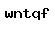## PDO数据库操作类——查询获取数据的实现

[文章作者：磨延城 转载请注明原文出处： https://mo2g.com/view/80/ ]

mPHP核心框架使用PDO数据库抽象层查询数据库中的数据,都是通过PDO的query()方法,如果你熟悉Mysql数据库的sql语句,那么理解起来就更轻松了,你完全可以把它当作mysql的query()来使用.

mPHP核心框架使用PDO数据库抽象层查询数据库中的数据，都是通过PDO的query()方法，如果你熟悉Mysql数据库的sql语句，那么理解起来就更轻松了，你完全可以把它当作mysql的query()来使用。

 id name 1 PDO 2 mPHP

```\$strSql = "select * from mo2g_test where id = 1";
mysql_query(\$strSql);
\$arrData = mysql_fetch_array();//获取找到的数据
print_r(\$arrData);//输出找到的数据```

PDO的查询方法如下

```\$strSql = "select * from mo2g_test where id = 1";
\$pdo->query(\$strSql);//返回影响了多少行数据
\$arrData = \$pdo->fetch();//获取找到的数据
print_r(\$arrData);//输出找到的数据```

```\$pdo->select('*','mo2g_test','id=1');//获取id为1的数据
\$arrData = \$pdo->fetch();//获取找到的数据
print_r(\$arrData);//输出找到的数据

\$pdo->select('*','mo2g_test','id>1','id');//根据id从大到小进行排序
\$pdo->select('*','mo2g_test','id>1','id asc');//根据id从小到大进行排序
\$pdo->select('*','mo2g_test','id>1',array('field'=>'id','order'=>'asc'));//根据id从小到大进行排序
\$pdo->select('*','mo2g_test','id>1','id','limit 1');//根据id从大到小进行排序,并只获取1条数据
\$arrData = \$pdo->fetch_all();//获取找到的所有数据
print_r(\$arrData);//输出找到的数据

//推荐使用的查询方式
public function query(\$strSql) {
\$this->result = \$this->db->query(\$strSql);
return \$this;
}

/*
\$select：要获取的属性
\$table：数据表名称
\$condition：获取条件
\$order：数据排序
\$limit：获取几条数据
*/
public function select(\$select,\$table,\$condition = '',\$order = array(), \$limit = '') {
if( is_array(\$order) && isset(\$order['order']) && ( \$order['order'] == 'desc' || \$order['order'] == 'asc' ) && isset(\$order['field']) ) {
\$order = "order by \$order[field] \$order[order]";
} elseif( is_string(\$order) ) {
\$order = "order by {\$order}";
} else \$order = '';
\$this->sql = \$strSql = \$condition == '' ?
"select \$select
from \$table
\$order
\$limit"
:
"select \$select
from \$table
where \$condition
\$order
\$limit";
if(\$this->result = \$this->db->query(\$strSql)) {
return \$this;
}
return \$this;
}
//获取所有查询到的数据
public function fetch_all() {
\$arrData = array();
if( \$this->result ) {
while(\$row = \$this->result->fetch(PDO::FETCH_ASSOC) ) {
\$arrData[] = \$row;
}
}
return \$arrData;
}
//获取一条查询到的数据
public function fetch() {
\$arrData = array();
if( \$this->result ) {
\$arrData = \$this->result->fetch(PDO::FETCH_ASSOC);
}
return \$arrData;
}```

1. 暂无评论...
2. ### 我来说两句:

切换

磨途歌检测发现,您当前使用的浏览器版本过低,要想使用画板模式,请先更新浏览器

切换